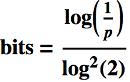I've spent a few days this week working on a side-project that relies heavily on Bloom Filters (look for a post on the result of my labours in the next week or so). If you don't know what a Bloom filter is, you should probably find out - they're very neat and have a huge range of fascinating applications.

I often need to do rough back-of-the-envelope reasoning about things, and I find that doing a bit of work to develop an intuition for how a new technique performs is usually worthwhile. So, here are three broad rules of thumb to remember when discussing Bloom filters down the pub:

## 1 - One byte per item in the input set gives about a 2% false positive rate.

In other words, we can add 1024 elements to a 1KB Bloom Filter, and check for set membership with about a 2% false positive rate. Nifty. Here are some common false positive rates and the approximate required bits per element, assuming an optimal choice of the number of hashes:

fp rate bits
50% 1.44
10% 4.79
2% 8.14
1% 9.58
0.1% 14.38
0.01% 19.17

Graphically, the relation between bits per element and the false positive rate when using an optimal number of hashes looks like this: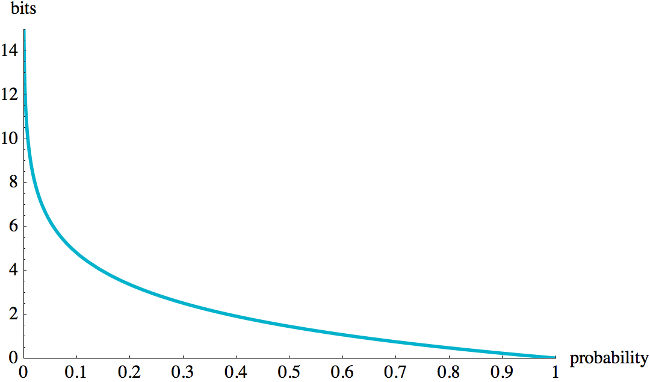Bits per element vs. false positive probability

## 2 - The optimal number of hash functions is about 0.7 times the number of bits per item.

This means that the number of hashes is "small", varying from about 3 at a 10% false positive rate, to about 13 at a 0.01% false positive rate.

## 3 - The number of hashes dominates performance.

The number of hashes determines the number of bits that need to be read to test for membership, the number of bits that need to be written to add an element, and the amount of computation needed to calculate hashes themselves. We may sometimes choose to use a less than optimal number of hashes for performance reasons (especially when we choose to round down when the calculated optimal number of hashes is fractional).

# The maths

Let's do some maths to justify the above, starting with two well-known results about Bloom filters that can be found in every description of the data structure. First, by a combinatoric argument we can show that the probability p of a false positive is approximated by the following formula, where k is the number of hash functions, n is the size of the input set and m is the size of the Bloom filter in bits: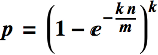Second, we know that k is optimal when: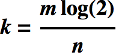Notice that in this formula, m/n is the number of bits per element in the Bloom filter. So, the optimal number of hashes grows linearly with the number of bits per element (b):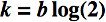Assuming an optimal choice for k in the first formula, we get :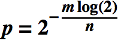Solving for m: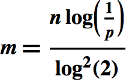It's clear from the above that for a given false-positive rate, the number of bits in a Bloom filter grows linearly with n. If we set n = 1, we get the following expression for the approximate number of bits needed per set element: